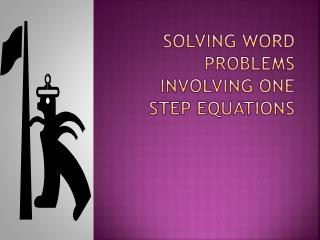# Solving word problems involving one step Equations - PowerPoint PPT PresentationDownload PresentationSolving word problems involving one step Equations

Solving word problems involving one step EquationsDownload Presentation## Solving word problems involving one step Equations

- - - - - - - - - - - - - - - - - - - - - - - - - - - E N D - - - - - - - - - - - - - - - - - - - - - - - - - - -
##### Presentation Transcript

1. Solving word problems involving one step Equations

2. Last week Danielle ran 17 miles more than Ryan. If Danielle ran 35 miles. how many miles did Ryan run? The miles Ryan ran plus seventeen equals miles Danielle ran Write in your own words Let R be the number of miles Ryan ran Define the variable Let 35 be the number of miles Danielle ran Write an equation R + 17 = 35 R + 17 = 35 -17 -17 add the opposite Solve the equation R = 18 Ryan ran 18 miles Write a sentence

3. In seven years Ted will be 22 years old. How old is he now? Age of Ted now plus seven equals Twenty two Write in your own words Let T be Ted’s age now Define the variable Let 22 be Ted’s age in seven years. Write an equation T + 7 = 22 T + 7 = 22 -7 -7 add the opposite Solve the equation T = 15 Ted now is 15 years old Write a sentence

4. The product of a number and three is twenty-seven. What is the number If I multiply a number by three the answer is 27 Write in your own words Define the variable Let n be the number 3 n = 27 Write an equation 3 n = 27 Solve the equation 3 3 divide by 3 n = 9 The number is 9 Write a sentence

5. A telephone calling card allows for 25 cents per minute plus one-time service charge of 75 cents. If the total cost of the card is \$5, find the number of minutes you can use the card Let m be the number of minutes Define the variable Let 75 be the one-time charge .25m +.75 = 5 Write an equation .25m + .75= 5 -.75 -.75 add the opposite Solve the equation .25m = 4.25 .25 .25 divide by 3 m = 17 Write a sentence The card can be used for 17minutes.